# Python Keywords

## Python Programming Training Certification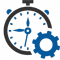Flexible Hours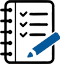100 Assignments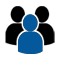Instructor Led online Training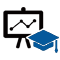50 LMS Access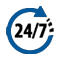24X7 Support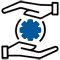100% Skill Level

### Enquire Now

4.9 out of 1000+ Ratings
Best Python Institute for Learning Python Course & Training, Live Project Training in Python with Django, Data Science and AI, Interview Assistance, Expert Coaching Trainers. Python Certification & Interview Assistance! Get free demo now!

### Course Overview

Python is one of the world’s top programming languages used today and Python training has become the most popular training across individuals. Training Basket’s Python Training & Certification course covers basic and advanced Python concepts and how to apply them in real-world applications.Python is a flexible and powerful open-source language that is easy to learn and consists of powerful libraries for data analysis and manipulation. Our Python training course content is curated by experts as per the standard Industry curriculum. The curriculum, coding challenges and real-life problems cover data operations in Python, strings, conditional statements, error handling, shell scripting, web scraping and the commonly used Python web framework Django. Take this Python training and certification course and become job-ready now.### Python Keywords

Python Keywords are special reserved words that convey a special meaning to the compiler/interpreter. Each keyword has a special meaning and a specific operation. These keywords can’t be used as a variable. Following is the List of Python Keywords.

 True False None and as asset def class continue break else finally elif del except global for if from import raise try or return pass nonlocal in not is lambda

Consider the following explanation of keywords.

• True – It represents the Boolean true, if the given condition is true, then it returns “True”. Non-zero values are treated as true.

False – It represents the Boolean false; if the given condition is false, then it returns “False”. Zero value is treated as false

None – It denotes the null value or void. An empty list or Zero can’t be treated as None.

and – It is a logical operator. It is used to check the multiple conditions. It returns true if both conditions are true. Consider the following truth table.

A B A and B
True True True
True False False
False True False
False False False
• r – It is a logical operator in Python. It returns true if one of the conditions is true. Consider the following truth
A B A and B
True True True
True False True
False True True
False False False
• not – It is a logical operator and inverts the truth value. Consider the following truth table.
A Not A
True False
False True
• assert – This keyword is used as the debugging tool in Python. It checks the correctness of the code. It raises an AssertionError if found any error in the code and also prints the message with an error.

Example:

• a = 10
b = 0
print(‘a is dividing by Zero’)
assert b != 0
print(a / b)

Output:

• a is dividing by Zero
Runtime Exception:
Traceback (most recent call last):
File “/home/40545678b342ce3b70beb1224bed345f.py”, line 4, in
assert b != 0, “Divide by 0 error”
AssertionError: Divide by 0 error
def –This keyword is used to declare the function in Python. If followed by the function name.
• def my_func(a,b):
c = a+b
print(c)
my_func(10,20)

Output:

• 30
class –It is used to represents the class in Python. The class is the blueprint of the objects. It is the collection of the variable and methods. Consider the following class.
• class Myclass:
#Variables……..
def function_name(self):
#statements………
continue –It is used to stop the execution of the current iteration. Consider the following example.
• a = 0
while a < 4:
a += 1
if a == 2:
continue
print(a)

Output:

• 1
3
4
break –It is used to terminate the loop execution and control transfer to the end of the loop. Consider the following example.

Example

• for i in range(5):
if(i==3):
break
print(i)
print(“End of execution”)

Output:

• 0
1
2
End of execution
If –It is used to represent the conditional statement. The execution of a particular block is decided by if statement. Consider the following example.

Example

• i = 18
if (1 < 12):
print(“I am less than 18”)

Output:

• I am less than 18
else –The else statement is used with the if statement. When if statement returns false, then else block is executed. Consider the following example.

Example

• n = 11
if(n%2 == 0):
print(“Even”)
else:
print(“odd”)

Output:

• Odd
elif –This Keyword is used to check the multiple conditions. It is short for else-if. If the previous condition is false, then check until the true condition is found. Condition the following example.

Example

• marks = int(input(“Enter the marks:”))
if(marks>=90):
print(“Excellent”)
elif(marks<90 and marks>=75):
print(“Very Good”)
elif(marks<75 and marks>=60):
print(“Good”)
else:
print(“Average”)

Output:

• Enter the marks:85
Very Good
del –It is used to delete the reference of the object. Consider the following example.

Example

• a=10
b=12
del a
print(b)
# a is no longer exist
print(a)

Output:

• 12
NameError: name ‘a’ is not defined
try, except –The try-except is used to handle the exceptions. The exceptions are run-time errors. Consider the following example.

Example

• a = 0
try:
b = 1/a
except Exception as e:
print(e)

Output:

• division by zero
raise –The raise keyword is used to through the exception forcefully. Consider the following example.

Example

• a = 5
if (a>2):
raise Exception(‘a should not exceed 2 ‘)

Output:

• Exception: a should not exceed 2
finally –The finally keyword is used to create a block of code that will always be executed no matter the else block raises an error or not. Consider the following example.

Example

• a=0
b=5
try:
c = b/a
print(c)
except Exception as e:
print(e)
finally:
print(‘Finally always executed’)

Output:

• division by zero
Finally always executed
for, while –Both keywords are used for iteration. The for keyword is used to iterate over the sequences (list, tuple, dictionary, string). A while loop is executed until the condition returns false. Consider the following example.

Example : For Loop

• list = [1,2,3,4,5]
for i in list:
print(i)

Output:

• 1
2
3
4
5

Example: While loop

• a = 0
while(a< 5):
print(a)
a = a+1 )

Output:

• 0
1
2
3
4
import – The import keyword is used to import modules in the current Python script. The module contains a runnable Python code.

Example

• import math
print(math.sqrt(25))

Output:

• 5
from – This keyword is used to import the specific function or attributes in the current Python script.

Example

• from math import sqrt
print(sqrt(25))

Output:

• 5
as – It is used to create a name alias. It provides the user-define name while importing a module.

Example

• import calendar as cal
print(cal.month_name)

Output:

• May
pass – The pass keyword is used to execute nothing or create a placeholder for future code. If we declare an empty class or function, it will through an error, so we use the pass keyword to declare an empty class or function.

Example

• class my_class:
pass

def my_func():
pass

return – The return keyword is used to return the result value or none to called function.

Example

• def sum(a,b):
c = a+b
return c

print(“The sum is:”,sum(25,15))

Output:

• The sum is: 40
is – This keyword is used to check if the two-variable refers to the same object. It returns the true if they refer to the same object otherwise false. Consider the following example.

Example

• x = 5
y = 5

a = []
b = []
print(x is y)
print(a is b)

Output:

• True
False
global – The global keyword is used to create a global variable inside the function. Any function can access the global. Consider the following example.

Example

• def my_func():
global a
a = 10
b = 20
c = a+b
print(c)

my_func()

def func():
print(a)

func()

Output:

• 30
10
nonlocal – The nonlocal is similar to the global and used to work with a variable inside the nested function(function inside a function). Consider the following example.

Example

• def outside_function():
a = 20
def inside_function():
nonlocal a
a = 30
print(“Inner function: “,a)
inside_function()
print(“Outer function: “,a)
outside_function()

Output:

• Inner function: 50
Outer function: 50
lambda – The lambda keyword is used to create the anonymous function in Python. It is an inline function without a name. Consider the following example.

Example

• a = lambda x: x**2
for i in range(1,6):
print(a(i))

Output:

• 1
4
9
16
25
yield – The yield keyword is used with the Python generator. It stops the function’s execution and returns value to the caller. Consider the following example.

Example

• def fun_Generator():
yield 1
yield 2
yield 3

# Driver code to check above generator function
for value in fun_Generator():
print(value)

Output:

• 1
2
3
with – The with keyword is used in the exception handling. It makes code cleaner and more readable. The advantage of using with, we don’t need to call close(). Consider the following example.

Example

• with open(‘file_path’, ‘w’) as file:
file.write(‘hello world !’)
None – The None keyword is used to define the null value. It is remembered that None does not indicate 0, false, or any empty data-types. It is an object of its data type, which is Consider the following example.

Example

• def return_none():
a = 10
b = 20
c = a + b

x = return_none()
print(x)

Output:

• None

We have covered all Python keywords. This is the brief introduction of Python Keywords. We will learn more in the upcoming tutorials.

### Recently Trained Students#### Jessica Biel

– Infosys

My instructor had sound Knowledge and used to puts a lot of effort that made the course as simple and easy as possible. I was aiming for with the help of the ZebLearn Online training imparted to me by this organization.#### Richard Harris

– ITC

I got my training from Zeblearn in the Python Certification Training, I would like to say that say he is one of the best trainers. He has not even trained me but also motivated me to explore more and the way he executed the project, in the end, was mind-blowing.

### Candidate’s Journey During Our Training Program#### Expert’s Advice & Selection of Module

Choosing the right type of module for the training is half the battle & Our Team of experts will help & guide you.#### Get Trained

Get Trained & Learn End to End Implementation from our Expert Trainer who are working on the same domain.#### Work on Projects

We Do make our student’s work on multiple case studies , scenario based tasks & projects in order to provide real-time exposure to them.#### Placements

We have a dedicated placement cell in order to provide placement assistance & relevant interviews to our candididates till selection